﻿ 三维弹性波方程的修正时空优化保辛数值求解方法
 地球物理学报2021, Vol. 64Issue (11): 4150-4165PDF

1. 清华大学数学科学系, 北京 100084;
2. 北京工商大学数学与统计学院应用统计系, 北京 100048;
3. 中国矿业大学(北京)理学院, 北京 100083

A modified time-space optimized symplectic method for solving 3D elastic wave equations
WANG Jian1, HE XiJun2, DONG XingPeng1, YANG DingHui1, LI JingShuang3, HUANG XueYuan2, ZHOU YanJie2
1. Department of Mathematical Sciences, Tsinghua University, Beijing 100084, China;
2. School of Mathematics and Statistics, Beijing Technology and Business University(BTBU), Beijing 100048, China;
3. School of Science, China University of Mining and Technology(Beijing), Beijing 100083, China
Abstract: Forward modeling is the basis of inversion research. In order to achieve full waveform inversion imaging based on 3D elastic wave equation, it is necessary to develop accurate and efficient 3D forward modeling methods with low numerical dispersion. For that reason, this paper combines the optimized finite difference operator with the modified symplectic partitioned Runge-Kutta (SPRK) scheme and extends it to 3D to develop a modified time-space optimized symplectic method (MTSOS) for solving the elastic wave equation in 3D inhomogeneous media. The method uses a second-order scheme to achieve third-order time accuracy, and is more suitable for solving the inhomogeneous medium model. The numerical dispersion error is smaller than the SPRK method, which improves the calculation accuracy. The numerical results show that the MTSOS method can give numerical simulation results accurately. The various phases in the inhomogeneous medium can be clearly seen, and there is no visible numerical dispersion, which shows the effectiveness of the new method.
Keywords: Numerical simulation    Three-dimensional elastic wave equation    Inhomogeneous medium
0 引言

1 修正时空优化保辛(MTSOS)方法 1.1 时间格式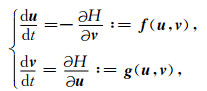(1)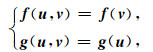(2)

fg分别是关于uv的线性函数，则称系统(1) 为线性可分的哈密尔顿系统.Qin和Zhang(1990)指出，波动方程是一个线性可分的哈密尔顿系统.对于弹性波方程：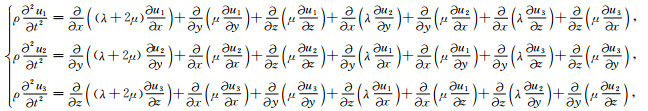(3)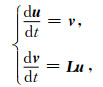(4)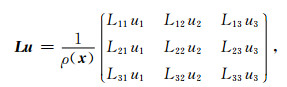(5)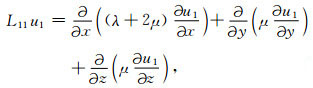(6)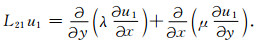(7)

Sanz-Serna(1988), s阶显式SPRK格式可表示为：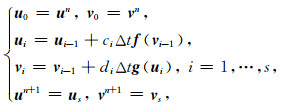(8)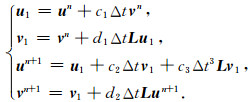(9)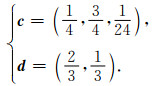(10)

1.2 空间格式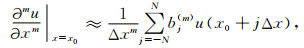(11)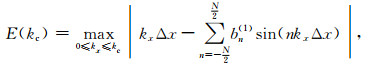(12)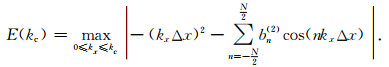(13)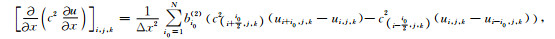(14)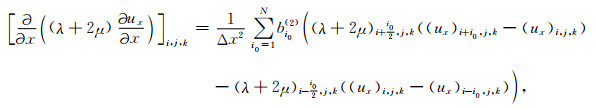(15)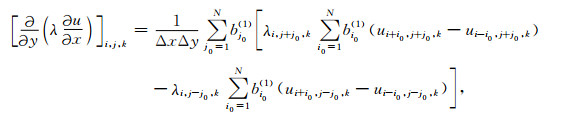(16)

1.3 等效介质参数与边界条件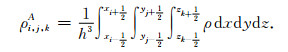(17)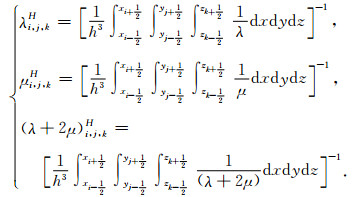(18)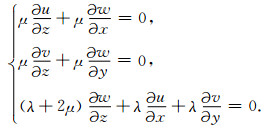(19)

z=0对应k=1，边界修正法首先引入一个虚拟层k=0，通过数值离散自由地表边界条件(19)来得到虚拟层的波场.具体来说，用如下格式来离散自由地表边界条件(Nilsson et al., 2007)：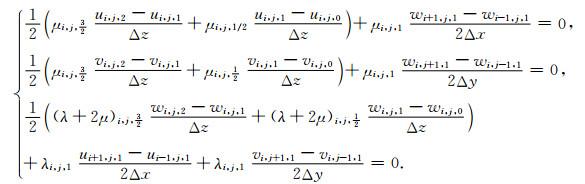(20)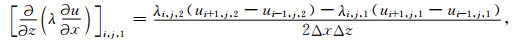(21)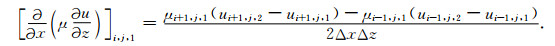(22)

2 三维MTSOS方法的稳定性和数值频散分析 2.1 稳定性条件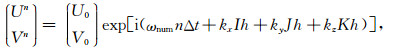(23)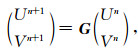(24)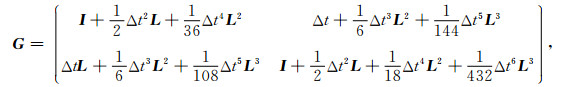(25)

I表示单位算子，L表示空间离散算子.设矩阵G的特征值为ψ，算子L的特征值为χ.由于波动方程是双曲型偏微分方程，故其空间离散算子χ＜0.由式(25)，ψ的特征多项式f(ψ)和χ满足关系：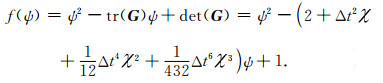(26)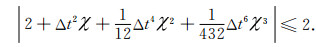(27)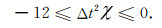(28)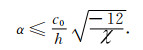(29)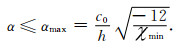(30)表 1 三维情形下不同空间精度的MTSOS方法与TSOS方法的最大库朗数 Table 1 Maximum Courant numbers of MTSOS method and TSOS method with different spatial accuracies in 3D case

2.2 数值频散分析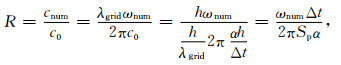(31)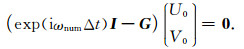(32)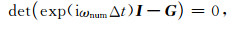(33)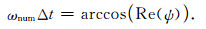(34)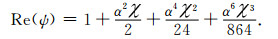(35)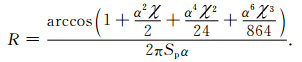(36)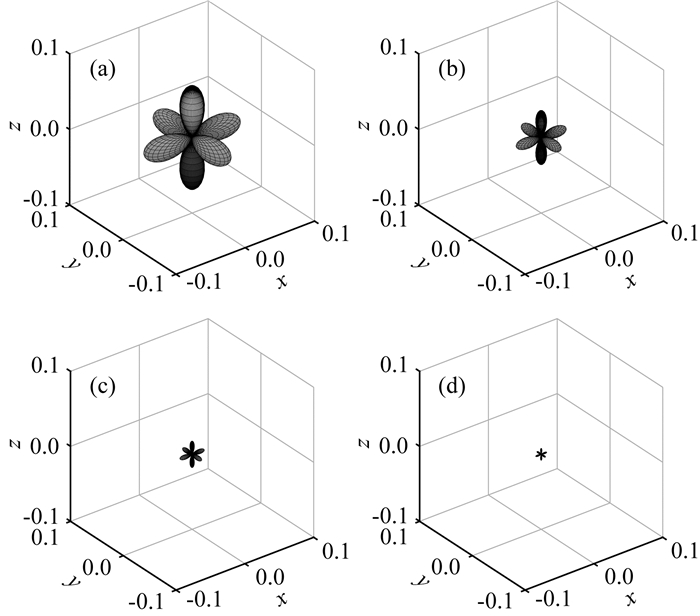图 1 不同空间精度的MTSOS方法在α=0.1, Sp=0.4时不同方向的数值频散误差 (a) 六阶；(b) 八阶；(c) 十阶；(d) 十二阶. Fig. 1 The numerical dispersion error of the MTSOS method with different spatial accuracies in different directions when α=0.1, Sp=0.4 (a) Sixth order; (b) Eighth order; (c) Tenth order; (d) Twelfth order.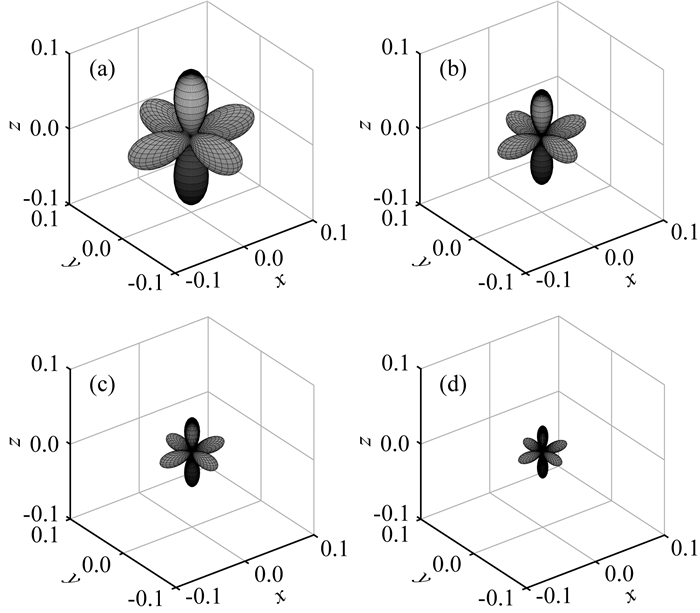图 2 不同空间精度的SPRK方法在α=0.1, Sp=0.4时不同方向的数值频散误差 (a) 六阶；(b) 八阶；(c) 十阶；(d) 十二阶. Fig. 2 The numerical dispersion error of the SPRK method with different spatial accuracies in different directions when α=0.1, Sp=0.4 (a) Sixth order; (b) Eighth order; (c) Tenth order; (d) Twelfth order.表 2 不同空间精度的MTSOS方法及SPRK方法在α=0.1, Sp=0.4时的最大数值频散误差 Table 2 The maximum numerical dispersion error of MTSOS method and SPRK method with different spatial accuracies when α=0.1, Sp=0.4
3 数值算例

3.1 均匀介质模型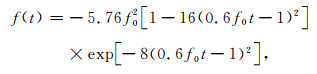(37)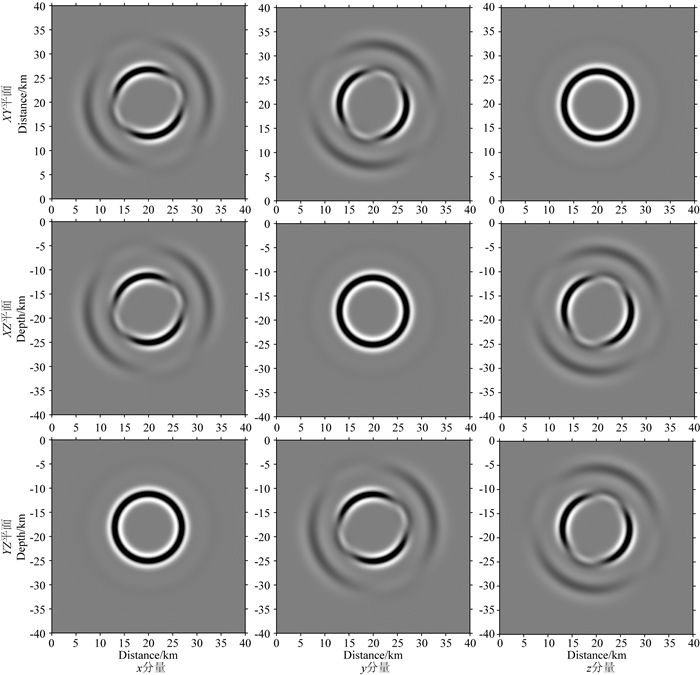图 3 MTSOS方法计算得到的t=3.0 s时刻位移场的不同分量分别在震源所在的XY、XZ和YZ平面的波场快照 Fig. 3 Snapshots of different components of the displacement field at the time t=3.0 s calculated by the MTSOS method in the XY, XZ and YZ planes where the seismic source is located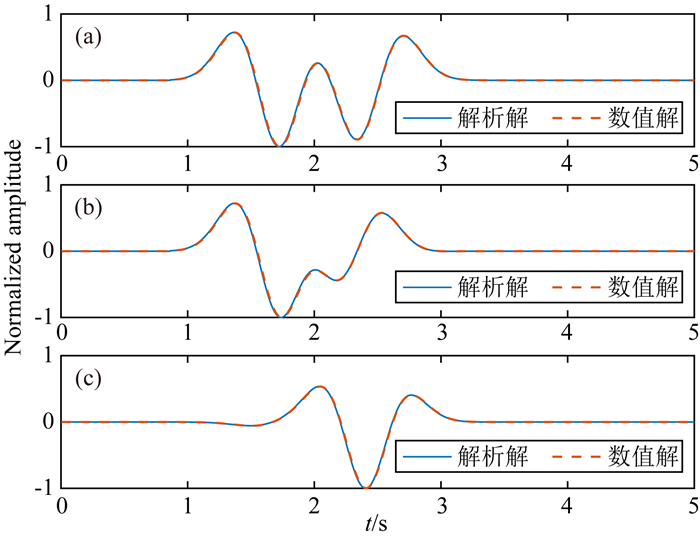图 4 六阶MTSOS方法计算得到的位于(23 km, 24 km, 18 km)处的接收器的波形图 (a) x分量；(b) y分量；(c) z分量. Fig. 4 Waveforms of the receiver located at (23 km, 24 km, 18 km) calculated by the sixth-order MTSOS method (a) x component; (b) y component; (c) z component.
3.2 双层介质模型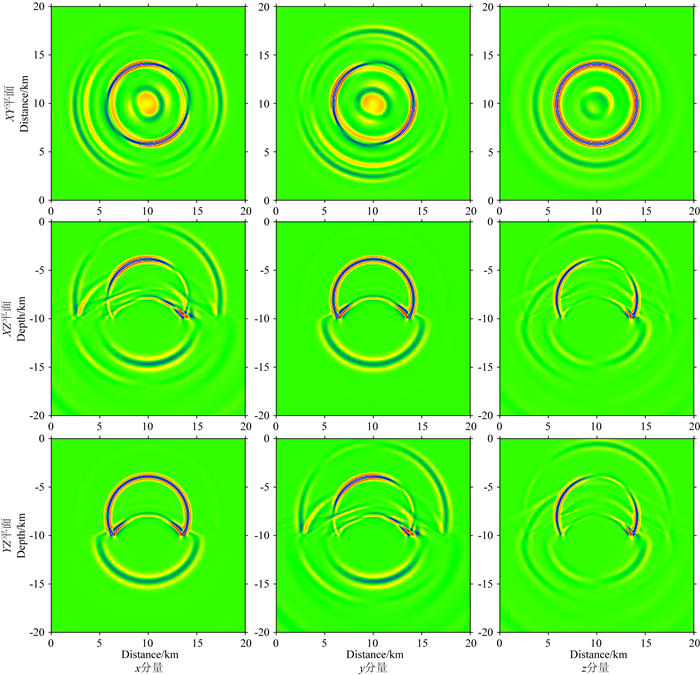图 5 六阶MTSOS方法计算得到的t=1.75 s时刻位移场的不同分量分别在震源所在的XY、XZ和YZ平面的波场快照 Fig. 5 The wave field snapshots of different components of the displacement field at the time t=1.75 s calculated by the sixth-order MTSOS method in the XY, XZ, and YZ planes where the seismic source is located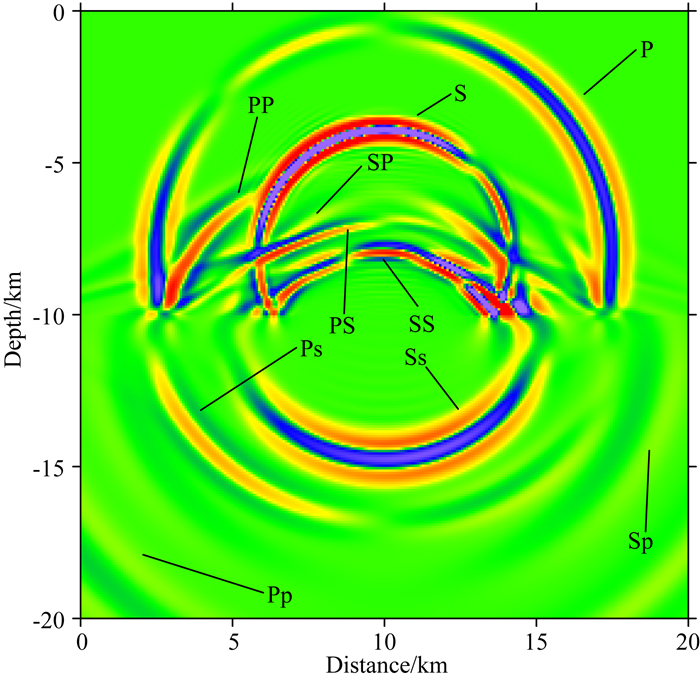图 6 六阶MTSOS方法计算得到的t=1.75 s时刻位移场的x分量在震源所在的XZ平面的波场快照 Fig. 6 The wave field snapshots of the x component of the displacement field at the time t=1.75 s calculated by the sixth-order MTSOS method in the XZ plane where the seismic source is located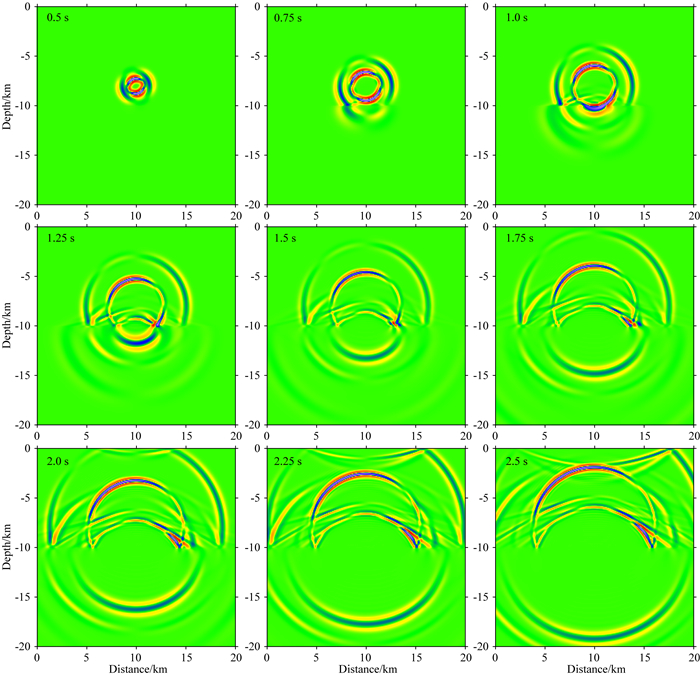图 7 六阶MTSOS方法计算得到的位移场的x分量在XZ平面上从t=0.5 s至t=2.5 s时刻的波场快照 Fig. 7 The wave field snapshots of the x component of the displacement field on the XZ plane from t=0.5 s to t=2.5 s calculated by the sixth-order MTSOS method
3.3 含水裂隙介质模型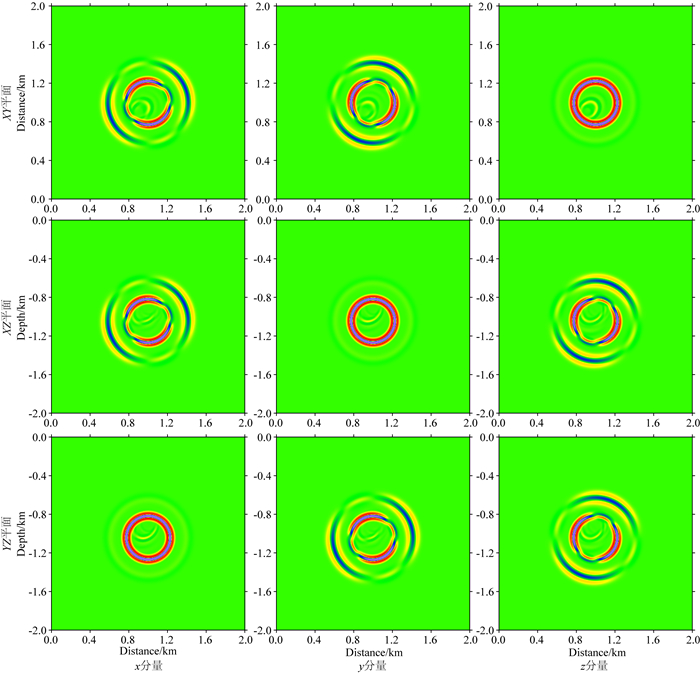图 8 六阶MTSOS方法计算得到的t=0.25 s时刻位移场的不同分量分别在震源所在的XY、XZ及YZ平面的波场快照 Fig. 8 The wave field snapshots of the different components of the displacement field at the time t=0.25 s calculated by the sixth-order MTSOS method in the XY, XZ, and YZ planes where the seismic source is located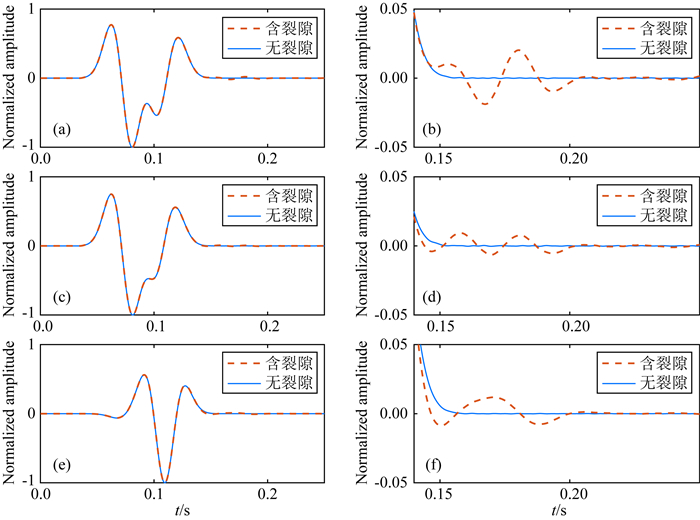图 9 六阶MTSOS方法计算得到的位于(0.925 km, 0.92 km, 1 km)接收器的波形图 (a) x分量；(b) x分量局部放大图；(c) y分量；(d) y分量局部放大图；(e) z分量；(f) z分量局部放大图. Fig. 9 Waveforms at the receiver located at (0.925 km, 0.92 km, 1 km) calculated by the sixth-order MTSOS method (a) x component; (b) Partial enlarged view of x component; (c) y component; (d) Partial enlarged view of y component; (e) z component; (f) Partial enlarged view of z component.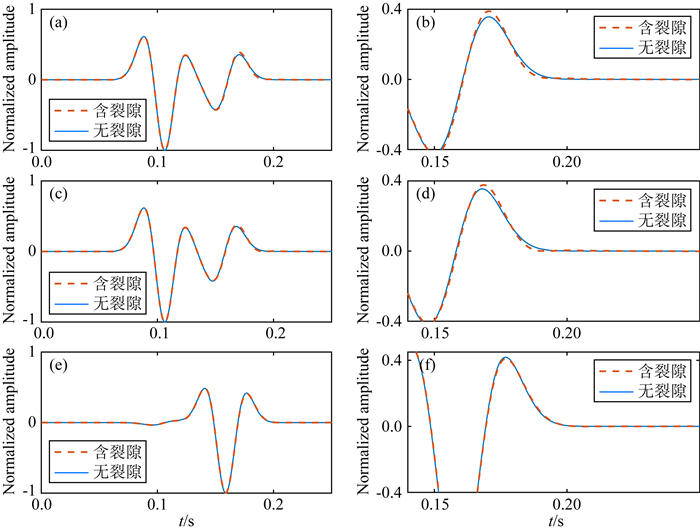图 10 六阶MTSOS方法计算得到的位于(0.885 km, 0.88 km, 1 km)接收器的波形图 (a) x分量；(b) x分量局部放大图；(c) y分量；(d) y分量局部放大图；(e) z分量；(f) z分量局部放大图. Fig. 10 Waveforms at the receiver located at (0.885 km, 0.88 km, 1 km) calculated by the sixth-order MTSOS method (a) x component; (b)Partial enlarged view of x component; (c) y component; (d) Partial enlarged view of y component; (e) z component; (f) Partial enlarged view of z component.
4 结论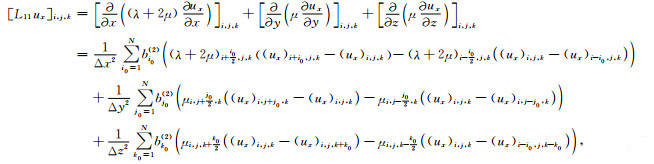(A1)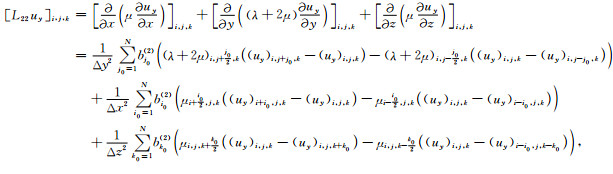(A2)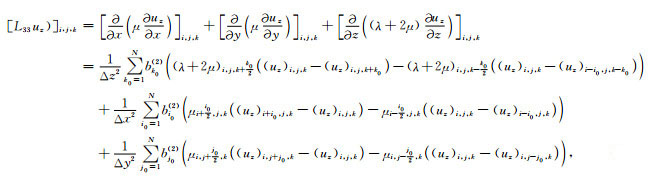(A3)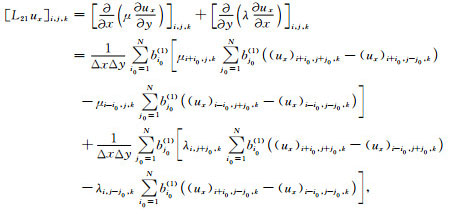(A4)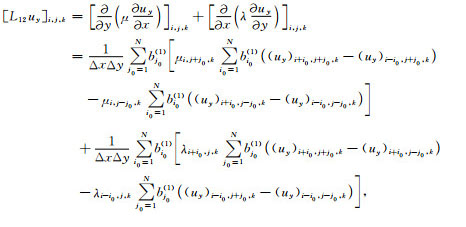(A5)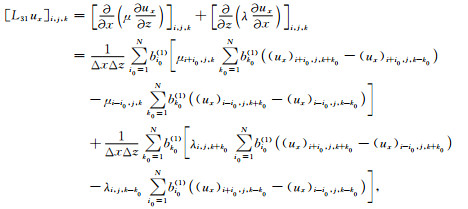(A6)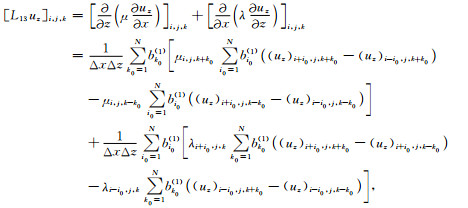(A7)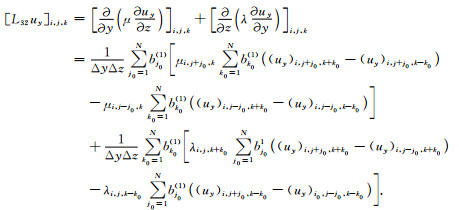(A8)

References
 Alterman Z, Karal F C Jr. 1969. Propagation of elastic waves in layered media by finite difference methods. Bulletin of the Seismological Society of America, 59(1): 471. DOI:10.1785/BSSA0590010471 Berenger J P. 1994. A perfectly matched layer for the absorption of electromagnetic waves. Journal of Computational Physics, 114(2): 185-200. DOI:10.1006/jcph.1994.1159 Clayton R, Engquist B. 1977. Absorbing boundary conditions for acoustic and elastic wave equations. Bulletin of the Seismological Society of America, 67(6): 1529-1540. DOI:10.1785/BSSA0670061529 Dablain M A. 1986. The application of high-order differencing to the scalar wave equation. Geophysics, 51(1): 54-66. DOI:10.1190/1.1442040 Fei T, Larner K. 1995. Elimination of numerical dispersion in finite-difference modeling and migration by flux-corrected transport. Geophysics, 60(6): 1830-1842. DOI:10.1190/1.1443915 Feng K, Qin M Z. 1987. The symplectic methods for the computation of Hamiltonian equations. //Zhu Y I, Guo B eds. Numerical Methods for Partial Differential Equations. Berlin: Springer, 1-37. Feng K, Qin M Z. 2003. Symplectic Geometric Algorithms for Hamiltonian Systems (in Chinese). Hangzhou: Zhejiang Science and Technology Press. Haras Z, Ta'asan S. 1994. Finite difference schemes for long-time integration. Journal of Computational Physics, 114(2): 265-279. DOI:10.1006/jcph.1994.1165 He X J, Yang D H, Ma X, et al. 2020. A modified numerical-flux-based discontinuous Galerkin method for 2D wave propagations in isotropic and anisotropic media. Geophysics, 85(5): T257-T273. DOI:10.1190/geo2019-0485.1 Kim J W, Lee D J. 1996. Optimized compact finite difference schemes with maximum resolution. AIAA Journal, 34(5): 887-893. DOI:10.2514/3.13164 Kirkpatrick S, Gelatt C D, Vecchi M P. 1983. Optimization by simulated annealing. Science, 220(4598): 671-680. DOI:10.1126/science.220.4598.671 Kosloff D D, Baysal E. 1982. Forward modeling by a Fourier method. Geophysics, 47(10): 1402-1412. DOI:10.1190/1.1441288 Lan H Q, Zhang Z, Xu T, et al. 2012. Effects due to the anisotropic stretching of the surface-fitting grid on the traveltime computation for irregular surface by the coordinate transforming method. Chinese Journal of Geophysics (in Chinese), 55(10): 3355-3369. DOI:10.6038/j.issn.0001-5733.2012.10.018 Lee C, Seo Y. 2002. A new compact spectral scheme for turbulence simulations. Journal of Computational Physics, 183(2): 438-469. DOI:10.1006/jcph.2002.7201 Liu S L, Yang D H, Ma J. 2017. A modified symplectic PRK scheme for seismic wave modeling. Computers & Geosciences, 99: 28-36. Lu J F, Guan Z. 2004. Numerical Solution of Partial Differential Equations (in Chinese). 2nd ed. Beijing: Tsinghua University Press. Lysmer J, Drake L A. 1972. A finite element method for seismology. Methods in Computational Physics: Advances in Research and Applications, 11: 181-216. Ma J, Yang D H, Tong P, et al. 2018. TSOS and TSOS-FK hybrid methods for modelling the propagation of seismic waves. Geophysical Journal International, 214(3): 1665-1682. DOI:10.1093/gji/ggy215 Ma X, Yang D H, Zhang J H. 2010. Symplectic partitioned Runge-Kutta method for solving the acoustic wave equation. Chinese Journal of Geophysics (in Chinese), 53(8): 1993-2003. DOI:10.3969/j.issn.0001-5733.2010.08.026 Ma X, Yang D H, Liu F Q. 2011. A nearly analytic symplectically partitioned Runge-Kutta method for 2-D seismic wave equations. Geophysical Journal International, 187(1): 480-496. DOI:10.1111/j.1365-246X.2011.05158.x Ma X, Yang D H, Song G J, et al. 2014. A low-dispersive symplectic partitioned Runge-Kutta method for solving seismic-wave equations: Ⅰ. scheme and theoretical analysis. Bulletin of the Seismological Society of America, 104(5): 2206-2225. DOI:10.1785/0120120210 Ma X, Yang D H. 2017. A phase-preserving and low-dispersive symplectic partitioned Runge-Kutta method for solving seismic wave equations. Geophysical Journal International, 209(3): 1534-1557. DOI:10.1093/gji/ggx097 Martin R, Komatitsch D, Gedney S D, et al. 2010. A high-order time and space formulation of the unsplit perfectly matched layer for the seismic wave equation using Auxiliary Differential Equations (ADE-PML). Computer Modeling in Engineering and Sciences, 56(1): 17. Moczo P, Kristek J, Vavryčuk V, et al. 2002. 3D heterogeneous staggered-grid finite-difference modeling of seismic motion with volume harmonic and arithmetic averaging of elastic moduli and densities. Bulletin of the Seismological Society of America, 92(8): 3042-3066. DOI:10.1785/0120010167 Nilsson S, Petersson N A, Sjögreen B, et al. 2007. Stable difference approximations for the elastic wave equation in second order formulation. SIAM Journal on Numerical Analysis, 45(5): 1902-1936. DOI:10.1137/060663520 Okunbor D, Skeel R D. 1992. Explicit canonical methods for Hamiltonian systems. Mathematics of Computation, 59(200): 439-455. DOI:10.1090/S0025-5718-1992-1136225-3 Priolo E, Seriani G. 1991. A numerical investigation of Chebyshev spectral element method for acoustic wave propagation. //13th World Congress Computation and Applied Mathematics, 2: 551-556. Qin M Z, Zhang M Q. 1990. Multi-stage symplectic schemes of two kinds of Hamiltonian systems for wave equations. Computers & Mathematics with Applications, 19(10): 51-62. Sanz-Serna J M. 1988. Runge-Kutta schemes for Hamiltonian systems. BIT Numerical Mathematics, 28(4): 877-883. DOI:10.1007/BF01954907 Schmid R. 1987. Infinite dimensional Hamiltonian systems. //Monographs and Textbooks in Physical Sciences. Biblionopolis. Sen M K, Stoffa P L. 2013. Global Optimization Methods in Geophysical Inversion. Cambridge: Cambridge University Press. Smith W D. 1975. The application of finite element analysis to body wave propagation problems. Geophysical Journal International, 42(2): 747-768. Wang J, Yang D H, Jing H, et al. 2019. Full waveform inversion based on the ensemble Kalman filter method using uniform sampling without replacement. Science Bulletin, 64(5): 321-330. DOI:10.1016/j.scib.2019.01.021 Wang L, Yang D H, Deng X Y. 2009. A WNAD method for seismic stress-field modeling in heterogeneous media. Chinese Journal of Geophysics (in Chinese), 52(6): 1526-1535. DOI:10.3969/j.issn.0001-5733.2009.06.014 Yang D H, Teng J W, Zhang Z J, et al. 2003. A nearly analytic discrete method for acoustic and elastic wave equations in anisotropic media. Bulletin of the Seismological Society of America, 93(2): 882-890. DOI:10.1785/0120020125 Yang D H, Peng J M, Lu M, et al. 2006. Optimal nearly analytic discrete approximation to the scalar wave equation. Bulletin of the Seismological Society of America, 96(3): 1114-1130. DOI:10.1785/0120050080 Yang D H, Song G J, Hua B L, et al. 2010. Simulation of acoustic wavefields in heterogeneous media: A robust method for automatic suppression of numerical dispersion. Geophysics, 75(3): T99-T110. DOI:10.1190/1.3428483 Zhang J H, Yao Z X. 2013. Optimized explicit finite-difference schemes for spatial derivatives using maximum norm. Journal of Computational Physics, 250: 511-526. DOI:10.1016/j.jcp.2013.04.029 冯康, 秦孟兆. 2003. 哈密尔顿系统的辛几何算法. 杭州: 浙江科技出版社. 兰海强, 张智, 徐涛, 等. 2012. 贴体网格各向异性对坐标变换法求解起伏地表下地震初至波走时的影响. 地球物理学报, 55(10): 3355-3369. DOI:10.6038/j.issn.0001-5733.2012.10.018 陆金甫, 关治. 2004. 偏微分方程数值解法. 2版. 北京: 清华大学出版社. 马啸, 杨顶辉, 张锦华. 2010. 求解声波方程的辛可分Runge-Kutta方法. 地球物理学报, 53(8): 1993-2003. DOI:10.3969/j.issn.0001-5733.2010.08.026 王磊, 杨顶辉, 邓小英. 2009. 非均匀介质中地震波应力场的WNAD方法及其数值模拟. 地球物理学报, 52(6): 1526-1535. DOI:10.3969/j.issn.0001-5733.2009.06.014